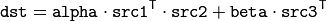# Operations on Matrices¶

## gpu::gemm¶

Performs generalized matrix multiplication.

C++: void gpu::gemm(const GpuMat& src1, const GpuMat& src2, double alpha, const GpuMat& src3, double beta, GpuMat& dst, int flags=0, Stream& stream=Stream::Null())
Parameters: src1 – First multiplied input matrix that should have CV_32FC1 , CV_64FC1 , CV_32FC2 , or CV_64FC2 type. src2 – Second multiplied input matrix of the same type as src1 . alpha – Weight of the matrix product. src3 – Third optional delta matrix added to the matrix product. It should have the same type as src1 and src2 . beta – Weight of src3 . dst – Destination matrix. It has the proper size and the same type as input matrices. flags – Operation flags: GEMM_1_T transpose src1 GEMM_2_T transpose src2 GEMM_3_T transpose src3 stream – Stream for the asynchronous version.

The function performs generalized matrix multiplication similar to the gemm functions in BLAS level 3. For example, gemm(src1, src2, alpha, src3, beta, dst, GEMM_1_T + GEMM_3_T) corresponds toNote

Transposition operation doesn’t support CV_64FC2 input type.

gemm()

## gpu::transpose¶

Transposes a matrix.

C++: void gpu::transpose(const GpuMat& src1, GpuMat& dst, Stream& stream=Stream::Null() )
Parameters: src1 – Source matrix. 1-, 4-, 8-byte element sizes are supported for now (CV_8UC1, CV_8UC4, CV_16UC2, CV_32FC1, etc). dst – Destination matrix. stream – Stream for the asynchronous version.

transpose()

## gpu::flip¶

Flips a 2D matrix around vertical, horizontal, or both axes.

C++: void gpu::flip(const GpuMat& a, GpuMat& b, int flipCode, Stream& stream=Stream::Null() )
Parameters: a – Source matrix. Supports 1, 3 and 4 channels images with CV_8U, CV_16U, CV_32S or CV_32F depth. b – Destination matrix. flipCode – Flip mode for the source: 0 Flips around x-axis. >0 Flips around y-axis. <0 Flips around both axes. stream – Stream for the asynchronous version.

flip()

## gpu::LUT¶

Transforms the source matrix into the destination matrix using the given look-up table: dst(I) = lut(src(I))

C++: void gpu::LUT(const GpuMat& src, const Mat& lut, GpuMat& dst, Stream& stream=Stream::Null())
Parameters: src – Source matrix. CV_8UC1 and CV_8UC3 matrices are supported for now. lut – Look-up table of 256 elements. It is a continuous CV_8U matrix. dst – Destination matrix with the same depth as lut and the same number of channels as src . stream – Stream for the asynchronous version.

LUT()

## gpu::merge¶

Makes a multi-channel matrix out of several single-channel matrices.

C++: void gpu::merge(const GpuMat* src, size_t n, GpuMat& dst, Stream& stream=Stream::Null())
C++: void gpu::merge(const vector<GpuMat>& src, GpuMat& dst, Stream& stream=Stream::Null())
Parameters: src – Array/vector of source matrices. n – Number of source matrices. dst – Destination matrix. stream – Stream for the asynchronous version.

merge()

## gpu::split¶

Copies each plane of a multi-channel matrix into an array.

C++: void gpu::split(const GpuMat& src, GpuMat* dst, Stream& stream=Stream::Null())
C++: void gpu::split(const GpuMat& src, vector<GpuMat>& dst, Stream& stream=Stream::Null())
Parameters: src – Source matrix. dst – Destination array/vector of single-channel matrices. stream – Stream for the asynchronous version.

split()

## gpu::magnitude¶

Computes magnitudes of complex matrix elements.

C++: void gpu::magnitude(const GpuMat& xy, GpuMat& magnitude, Stream& stream=Stream::Null() )
C++: void gpu::magnitude(const GpuMat& x, const GpuMat& y, GpuMat& magnitude, Stream& stream=Stream::Null())
Parameters: xy – Source complex matrix in the interleaved format ( CV_32FC2 ). x – Source matrix containing real components ( CV_32FC1 ). y – Source matrix containing imaginary components ( CV_32FC1 ). magnitude – Destination matrix of float magnitudes ( CV_32FC1 ). stream – Stream for the asynchronous version.

magnitude()

## gpu::magnitudeSqr¶

Computes squared magnitudes of complex matrix elements.

C++: void gpu::magnitudeSqr(const GpuMat& xy, GpuMat& magnitude, Stream& stream=Stream::Null() )
C++: void gpu::magnitudeSqr(const GpuMat& x, const GpuMat& y, GpuMat& magnitude, Stream& stream=Stream::Null())
Parameters: xy – Source complex matrix in the interleaved format ( CV_32FC2 ). x – Source matrix containing real components ( CV_32FC1 ). y – Source matrix containing imaginary components ( CV_32FC1 ). magnitude – Destination matrix of float magnitude squares ( CV_32FC1 ). stream – Stream for the asynchronous version.

## gpu::phase¶

Computes polar angles of complex matrix elements.

C++: void gpu::phase(const GpuMat& x, const GpuMat& y, GpuMat& angle, bool angleInDegrees=false, Stream& stream=Stream::Null())
Parameters: x – Source matrix containing real components ( CV_32FC1 ). y – Source matrix containing imaginary components ( CV_32FC1 ). angle – Destination matrix of angles ( CV_32FC1 ). angleInDegrees – Flag for angles that must be evaluated in degrees. stream – Stream for the asynchronous version.

phase()

## gpu::cartToPolar¶

Converts Cartesian coordinates into polar.

C++: void gpu::cartToPolar(const GpuMat& x, const GpuMat& y, GpuMat& magnitude, GpuMat& angle, bool angleInDegrees=false, Stream& stream=Stream::Null())
Parameters: x – Source matrix containing real components ( CV_32FC1 ). y – Source matrix containing imaginary components ( CV_32FC1 ). magnitude – Destination matrix of float magnitudes ( CV_32FC1 ). angle – Destination matrix of angles ( CV_32FC1 ). angleInDegrees – Flag for angles that must be evaluated in degrees. stream – Stream for the asynchronous version.

cartToPolar()

## gpu::polarToCart¶

Converts polar coordinates into Cartesian.

C++: void gpu::polarToCart(const GpuMat& magnitude, const GpuMat& angle, GpuMat& x, GpuMat& y, bool angleInDegrees=false, Stream& stream=Stream::Null())
Parameters: magnitude – Source matrix containing magnitudes ( CV_32FC1 ). angle – Source matrix containing angles ( CV_32FC1 ). x – Destination matrix of real components ( CV_32FC1 ). y – Destination matrix of imaginary components ( CV_32FC1 ). angleInDegrees – Flag that indicates angles in degrees. stream – Stream for the asynchronous version.

polarToCart()